•• OpenStax
• OpenStax
$$\newcommand{\vecs}{\overset { \rightharpoonup} {\mathbf{#1}} }$$ $$\newcommand{\vecd}{\overset{-\!-\!\rightharpoonup}{\vphantom{a}\smash {#1}}}$$$$\newcommand{\id}{\mathrm{id}}$$ $$\newcommand{\Span}{\mathrm{span}}$$ $$\newcommand{\kernel}{\mathrm{null}\,}$$ $$\newcommand{\range}{\mathrm{range}\,}$$ $$\newcommand{\RealPart}{\mathrm{Re}}$$ $$\newcommand{\ImaginaryPart}{\mathrm{Im}}$$ $$\newcommand{\Argument}{\mathrm{Arg}}$$ $$\newcommand{\norm}{\| #1 \|}$$ $$\newcommand{\inner}{\langle #1, #2 \rangle}$$ $$\newcommand{\Span}{\mathrm{span}}$$ $$\newcommand{\id}{\mathrm{id}}$$ $$\newcommand{\Span}{\mathrm{span}}$$ $$\newcommand{\kernel}{\mathrm{null}\,}$$ $$\newcommand{\range}{\mathrm{range}\,}$$ $$\newcommand{\RealPart}{\mathrm{Re}}$$ $$\newcommand{\ImaginaryPart}{\mathrm{Im}}$$ $$\newcommand{\Argument}{\mathrm{Arg}}$$ $$\newcommand{\norm}{\| #1 \|}$$ $$\newcommand{\inner}{\langle #1, #2 \rangle}$$ $$\newcommand{\Span}{\mathrm{span}}$$$$\newcommand{\AA}{\unicode[.8,0]{x212B}}$$

##### Learning Objectives

By the end of this section, you will be able to:

• Use the discriminant to predict the number and type of solutions of a quadratic equation
• Identify the most appropriate method to use to solve a quadratic equation

Before you get started, take this readiness quiz.

1. Evaluate $$b^{2}-4 a b$$ when $$a=3$$ and $$b=−2$$.
2. Simplify $$\sqrt{108}$$.
3. Simplify $$\sqrt{50}$$.

When we solved quadratic equations in the last section by completing the square, we took the same steps every time. By the end of the exercise set, you may have been wondering ‘isn’t there an easier way to do this?’ The answer is ‘yes’. Mathematicians look for patterns when they do things over and over in order to make their work easier. In this section we will derive and use a formula to find the solution of a quadratic equation.

We have already seen how to solve a formula for a specific variable ‘in general’, so that we would do the algebraic steps only once, and then use the new formula to find the value of the specific variable. Now we will go through the steps of completing the square using the general form of a quadratic equation to solve a quadratic equation for $$x$$.

We start with the standard form of a quadratic equation and solve it for $$x$$ by completing the square.

 $$ax^2 + bx + c = 0, \quad a \ne 0$$ Isolate the variable terms on one side. $$ax^2 + bx \quad = -c$$ Make the coefficient of $$x^{2}$$ equal to $$1$$, by dividing by $$a$$. $$\dfrac{ax^2}{a} + \dfrac{b}{a}x \quad = -\dfrac{c}{a}$$ Simplify. $$x^2+ \dfrac{b}{a}x \quad = -\dfrac{c}{a}$$ To complete the square, find $$\left(\dfrac{1}{2} \cdot \dfrac{b}{a}\right)^{2}$$ and add it to both sides of the equation. $$\left(\dfrac{1}{2} \dfrac{b}{a}\right)^{2}=\dfrac{b^{2}}{4 a^{2}}$$ $$x^2 + \dfrac{b}{a}x +{\color{red}{\dfrac{b^2}{4a^2}}}{\color{black}{ = -\dfrac{c}{a}\,+\,}}{\color{red}{\dfrac{b^2}{4a^2}}}$$ The left side is a perfect square, factor it. $$\left( x + \dfrac{b}{2a}\right)^2 = -\dfrac{c}{a}+\dfrac{b^2}{4a^2}$$ Find the common denominator of the right side and write equivalent fractions with the common denominator. $$\left( x + \dfrac{b}{2a}\right)^2 =\dfrac{b^2}{4a^2}-\dfrac{c\cdot\color{red}{4a}}{a\cdot\color{red}{4a}}$$ Simplify. $$\left( x + \dfrac{b}{2a}\right)^2 =\dfrac{b^2}{4a^2}-\dfrac{4ac}{4a^2}$$ Combine to one fraction. $$\left( x + \dfrac{b}{2a}\right)^2 =\dfrac{b^2-4ac}{4a^2}$$ Use the square root property. $$x + \dfrac{b}{2a}= \pm\sqrt{\dfrac{b^2-4ac}{4a^2}}$$ Simplify the radical. $$x + \dfrac{b}{2a}= \pm\dfrac{\sqrt{b^2-4ac}}{2a}$$ Add $$-\dfrac{b}{2a}$$ to both sides of the equation. $$x = -\dfrac{b}{2a} \pm\dfrac{\sqrt{b^2-4ac}}{2a}$$ Combine the terms on the right side. $$x = \dfrac{-b\pm\sqrt{b^2-4ac}}{2a}$$

The final equation is called the "Quadratic Formula."

##### Definition $$\PageIndex{1}$$: Quadratic Formula

The solutions to a quadratic equation of the form $$a x^{2}+b x+c=0$$, where $$a≠0$$ are given by the formula:

$x=\dfrac{-b \pm \sqrt{b^{2}-4 a c}}{2 a} \label{quad}$

To use the Quadratic Formula, we substitute the values of $$a,b$$, and $$c$$ from the standard form into the expression on the right side of the formula. Then we simplify the expression. The result is the pair of solutions to the quadratic equation.

Notice the Quadratic Formula (Equation \ref{quad}) is an equation. Make sure you use both sides of the equation.

##### Example $$\PageIndex{1}$$ How to Solve a Quadratic Equation Using the Quadratic Formula

Solve by using the Quadratic Formula: $$2 x^{2}+9 x-5=0$$.

Solution:

 Step 1: Write the quadratic equation in standard form. Identify the $$a,b,c$$ values. This equation is in standard form. \begin{aligned} \color{red}{a x^{2}+b x+c =0} \\ 2 x^{2}+9 x-5 =0 \\ a=2, b =9, c=-5 \end{aligned} Step 2: Write the quadratic formula. Then substitute in the values of $$a,b,c$$. Substitute in $$a=2, b=9, c=-5$$ $$x=\dfrac{-b \pm \sqrt{b^{2}-4 a c}}{2 a}$$ $$x=\dfrac{-9 \pm \sqrt{9^{2}-4 \cdot 2 \cdot(-5)}}{2 \cdot 2}$$ Step 3: Simplify the fraction, and solve for $$x$$. $$\begin{array}{l}{x=\dfrac{-9 \pm \sqrt{81-(-40)}}{4}} \\ {x=\dfrac{-9 \pm \sqrt{121}}{4}} \\ {x=\dfrac{-9 \pm 11}{4}} \\ {x=\dfrac{-9+11}{4}}\quad x=\dfrac{-9-11}{4} \\ {x=\dfrac{2}{4} \quad \quad\:\:\: x=\dfrac{-20}{4}}\\ {x=\dfrac{1}{2} \quad\quad\:\:\: x=-5}\end{array}$$ Step 4: Check the solutions. Put each answer in the original equation to check. Substitute $$x=\color{red}{\dfrac{1}{2}}$$ and $$x=\color{red}{-5}$$. \begin{aligned}2 x^{2}+9 x-5&=0 \\ 2\color{black}{\left(\color{red}{\dfrac{1}{2}}\right)}^{2}+9 \cdot \color{red}{\dfrac{1}{2}}\color{black}{-}5 &\stackrel{?}{=} 0 \\ 2\cdot\dfrac{1}{4}+0\cdot\dfrac{1}{2}-5&\stackrel{?}{=}0 \\ 2\cdot\dfrac{1}{4}+9\cdot\dfrac{1}{2}-5&\stackrel{?}{=}0 \\ \dfrac{1}{2}+\dfrac{9}{2}-5&\stackrel{?}{=}0 \\ \dfrac{10}{2}-5&\stackrel{?}{=}0 \\5-5&\stackrel{?}{=}0\\0&=0\end{aligned} $$\begin{array}{r}{2 x^{2}+9 x-5=0} \\ {2(\color{red}{-5}\color{black}{)}^{2}+9(\color{red}{-5}\color{black}{)}-5\stackrel{?}{=}0} \\ {2 \cdot 25-45-5\stackrel{?}{=}0} \\ {50-45-5\stackrel{?}{=}0} \\ {0=0}\end{array}$$
##### Exercise $$\PageIndex{1}$$

Solve by using the Quadratic Formula: $$3 y^{2}-5 y+2=0$$.

$$y=1, y=\dfrac{2}{3}$$

##### Exercise $$\PageIndex{2}$$

Solve by using the Quadratic Formula: $$4 z^{2}+2 z-6=0$$.

$$z=1, z=-\dfrac{3}{2}$$

1. Write the quadratic equation in standard form, $$a x^{2}+b x+c=0$$. Identify the values of $$a,b$$, and $$c$$.
2. Write the Quadratic Formula. Then substitute in the values of $$a,b$$, and $$c$$.
3. Simplify.
4. Check the solutions.

If you say the formula as you write it in each problem, you’ll have it memorized in no time! And remember, the Quadratic Formula is an EQUATION. Be sure you start with “$$x=$$”.

##### Example $$\PageIndex{2}$$

Solve by using the Quadratic Formula: $$x^{2}-6 x=-5$$.

Solution:

 $$x^{2}-6 x=-5$$ Write the equation in standard form by adding $$5$$ to each side. $$x^{2}-6 x+5=0$$ This equation is now in standard form. $${\color{red}{\small{ax^2+bx + c} = \small{0}}}$$ $$x^2 - 6x + 5 = 0$$ Identify the values of $$\color{cyan}a$$, $$\color{red}b$$, $$\color{limegreen}c$$. $${\color{cyan}a=1}$$, $${\color{red}b=-6}$$, $${\color{limegreen}c=5}$$ Write the Quadratic Formula. $$x=\dfrac{-b \pm \sqrt{b^{2}-4 a c}}{2 a}$$ Then substitute in the values of $$a, b, c$$. $$x=\dfrac{-\color{red} (-6 ) \color{black} \pm \sqrt{\color{red}(-6) \color{black}^{2}-4 \cdot \color{cyan}1 \color{black} \cdot ( \color{limegreen}5 \color{black})}}{2 \cdot \color{cyan} 1}$$ Simplify. $$x=\dfrac{6 \pm \sqrt{36-20}}{2}$$ $$x=\dfrac{6 \pm \sqrt{16}}{2}$$ $$x=\dfrac{6 \pm 4}{2}$$ Rewrite to show two solutions. $$x=\frac{6+4}{2}, \quad x=\frac{6-4}{2}$$ Simplify. $$x=\frac{10}{2}, \quad x=\frac{2}{2}$$ $$x=5, \quad x=1$$ Check:##### Exercise $$\PageIndex{3}$$

Solve by using the Quadratic Formula: $$a^{2}-2 a=15$$.

$$a=-3, a=5$$

##### Exercise $$\PageIndex{4}$$

Solve by using the Quadratic Formula: $$b^{2}+24=-10 b$$.

$$b=-6, b=-4$$

When we solved quadratic equations by using the Square Root Property, we sometimes got answers that had radicals. That can happen, too, when using the Quadratic Formula. If we get a radical as a solution, the final answer must have the radical in its simplified form.

##### Example $$\PageIndex{3}$$

Solve by using the Quadratic Formula: $$2 x^{2}+10 x+11=0$$.

Solution:This equation is in standard form.Identify the values of $$a,b$$ and $$c$$.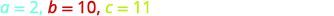Write the Quadratic Formula. $$x=\dfrac{-b \pm \sqrt{b^{2}-4 a c}}{2 a}$$ Then substitute in the values of $$a, b$$, and $$c$$.Simplify. $$x=\dfrac{-10 \pm \sqrt{100-88}}{4}$$ $$x=\dfrac{-10 \pm \sqrt{12}}{4}$$ Simplify the radical. $$x=\dfrac{-10 \pm 2 \sqrt{3}}{4}$$ Factor out the common factor in the numerator.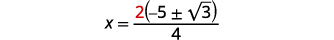$$x=\dfrac{\color{red}{2}(-5 \pm \sqrt{3})}{4}$$ Remove the common factors. $$x=\dfrac{-5 \pm \sqrt{3}}{2}$$ Rewrite to show two solutions. $$x=\dfrac{-5+\sqrt{3}}{2}, \quad x=\dfrac{-5-\sqrt{3}}{2}$$ Check: We leave the check for you!
##### Exercise $$\PageIndex{5}$$

Solve by using the Quadratic Formula: $$3 m^{2}+12 m+7=0$$.

$$m=\dfrac{-6+\sqrt{15}}{3}, m=\dfrac{-6-\sqrt{15}}{3}$$

##### Exercise $$\PageIndex{6}$$

Solve by using the Quadratic Formula: $$5 n^{2}+4 n-4=0$$.

$$n=\dfrac{-2+2 \sqrt{6}}{5}, n=\dfrac{-2-2 \sqrt{6}}{5}$$

When we substitute $$a, b$$, and $$c$$ into the Quadratic Formula and the radicand is negative, the quadratic equation will have imaginary or complex solutions. We will see this in the next example.

##### Example $$\PageIndex{4}$$

Solve by using the Quadratic Formula: $$3 p^{2}+2 p+9=0$$.

Solution:This equation is in standard form.Identify the values of $$a,b,c$$.Write the Quadratic Formula.Then substitute in the values of $$a,b,c$$.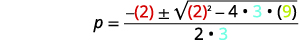Simplify.Simplify the radical using complex numbers.Simplify the radical.Factor the common factor in the numerator.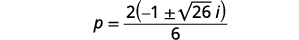Remove the common factors.Rewrite in standard $$a+bi$$ form.Write as two solutions.##### Exercise $$\PageIndex{7}$$

Solve by using the Quadratic Formula: $$4 a^{2}-2 a+8=0$$.

$$a=\dfrac{1}{4}+\dfrac{\sqrt{31}}{4} i, \quad a=\dfrac{1}{4}-\dfrac{\sqrt{31}}{4} i$$

##### Exercise $$\PageIndex{8}$$

Solve by using the Quadratic Formula: $$5 b^{2}+2 b+4=0$$.

$$b=-\dfrac{1}{5}+\dfrac{\sqrt{19}}{5} i, \quad b=-\dfrac{1}{5}-\dfrac{\sqrt{19}}{5} i$$

Remember, to use the Quadratic Formula, the equation must be written in standard form, $$a x^{2}+b x+c=0$$. Sometimes, we will need to do some algebra to get the equation into standard form before we can use the Quadratic Formula.

##### Example $$\PageIndex{5}$$

Solve by using the Quadratic Formula: $$x(x+6)+4=0$$.

Solution:

Our first step is to get the equation in standard form.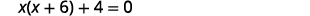Distribute to get the equation in standard form.This equation is now in standard form.Identify the values of $$a,b,c$$.Write the Quadratic Formula.Then substitute in the values of $$a,b,c$$.Simplify.Simplify the radical.Factor the common factor in the numerator.Remove the common factors.Write as two solutions.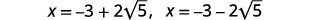Check: We leave the check for you!
##### Exercise $$\PageIndex{9}$$

Solve by using the Quadratic Formula: $$x(x+2)−5=0$$.

$$x=-1+\sqrt{6}, x=-1-\sqrt{6}$$

##### Exercise $$\PageIndex{10}$$

Solve by using the Quadratic Formula: $$3y(y−2)−3=0$$.

$$y=1+\sqrt{2}, y=1-\sqrt{2}$$

When we solved linear equations, if an equation had too many fractions we cleared the fractions by multiplying both sides of the equation by the LCD. This gave us an equivalent equation—without fractions— to solve. We can use the same strategy with quadratic equations.

##### Example $$\PageIndex{6}$$

Solve by using the Quadratic Formula: $$\dfrac{1}{2} u^{2}+\dfrac{2}{3} u=\dfrac{1}{3}$$.

Solution:

Our first step is to clear the fractions.Multiply both sides by the LCD, $$6$$, to clear the fractions.Multiply.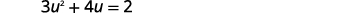Subtract $$2$$ to get the equation in standard form.Identify the values of $$a, b$$, and $$c$$.Write the Quadratic Formula.Then substitute in the values of $$a, b,$$ and $$c$$.Simplify.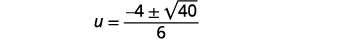Simplify the radical.Factor the common factor in the numerator.Remove the common factors.Rewrite to show two solutions.Check: We leave the check for you!
##### Exercise $$\PageIndex{11}$$

Solve by using the Quadratic Formula: $$\dfrac{1}{4} c^{2}-\dfrac{1}{3} c=\dfrac{1}{12}$$.

$$c=\dfrac{2+\sqrt{7}}{3}, \quad c=\dfrac{2-\sqrt{7}}{3}$$

##### Exercise $$\PageIndex{12}$$

Solve by using the Quadratic Formula: $$\dfrac{1}{9} d^{2}-\dfrac{1}{2} d=-\dfrac{1}{3}$$.

$$d=\dfrac{9+\sqrt{33}}{4}, d=\dfrac{9-\sqrt{33}}{4}$$

Think about the equation $$(x-3)^{2}=0$$. We know from the Zero Product Property that this equation has only one solution, $$x=3$$.

We will see in the next example how using the Quadratic Formula to solve an equation whose standard form is a perfect square trinomial equal to $$0$$ gives just one solution. Notice that once the radicand is simplified it becomes $$0$$, which leads to only one solution.

##### Example $$\PageIndex{7}$$

Solve by using the Quadratic Formula: $$4 x^{2}-20 x=-25$$.

Solution:Add $$25$$ to get the equation in standard form.Identify the values of $$a, b$$, and $$c$$.Write the quadratic formula.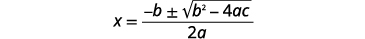Then substitute in the values of $$a, b$$, and $$c$$.Simplify.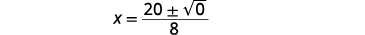Simplify the radical.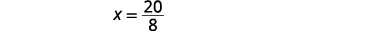Simplify the fraction.Check: We leave the check for you!

Did you recognize that $$4 x^{2}-20 x+25$$ is a perfect square trinomial. It is equivalent to $$(2 x-5)^{2}$$? If you solve $$4 x^{2}-20 x+25=0$$ by factoring and then using the Square Root Property, do you get the same result?

##### Exercise $$\PageIndex{13}$$

Solve by using the Quadratic Formula: $$r^{2}+10 r+25=0$$.

$$r=-5$$

##### Exercise $$\PageIndex{14}$$

Solve by using the Quadratic Formula: $$25 t^{2}-40 t=-16$$.

$$t=\dfrac{4}{5}$$

## Use the Discriminant to Predict the Number and Type of Solutions of a Quadratic Equation

When we solved the quadratic equations in the previous examples, sometimes we got two real solutions, one real solution, and sometimes two complex solutions. Is there a way to predict the number and type of solutions to a quadratic equation without actually solving the equation?

Yes, the expression under the radical of the Quadratic Formula makes it easy for us to determine the number and type of solutions. This expression is called the discriminant.

##### Definition $$\PageIndex{2}$$

Discriminant

Let’s look at the discriminant of the equations in some of the examples and the number and type of solutions to those quadratic equations.

Quadratic Equation (in standard form) Discriminat $$b^{2}-4ac$$ Value of the Discriminant Number and Type of Solutions
$$2 x^{2}+9 x-5=0$$ \begin{aligned} 9^{2}-& 4 \cdot 2(-5) \\ & 121 \end{aligned} $$+$$ $$2$$ real
$$4 x^{2}-20 x+25=0$$ $$(-20)^{2}-4 \cdot 4 \cdot 25$$
$$0$$
$$0$$ $$1$$ real
$$3 p^{2}+2 p+9=0$$ $$2^{2}-4 \cdot 3 \cdot 9$$
$$-104$$
$$-$$ $$2$$ complex
Table 9.3.9

### Using the Discriminant $$b^{2}-4ac$$, to Determine the Number and Type of Solutions of a Quadratic Equation

For a quadratic equation of the form $$ax^{2}+bx+c=0$$, $$a \neq 0$$,

• If $$b^{2}-4 a c>0$$, the equation has $$2$$ real solutions.
• if $$b^{2}-4 a c=0$$, the equation has $$1$$ real solution.
• if $$b^{2}-4 a c<0$$, the equation has $$2$$ complex solutions.
##### Example $$\PageIndex{8}$$

Determine the number of solutions to each quadratic equation.

1. $$3 x^{2}+7 x-9=0$$
2. $$5 n^{2}+n+4=0$$
3. $$9 y^{2}-6 y+1=0$$

Solution:

To determine the number of solutions of each quadratic equation, we will look at its discriminant.

a.

$$3 x^{2}+7 x-9=0$$

The equation is in standard form, identify $$a, b$$, and $$c$$.

$$a=3, \quad b=7, \quad c=-9$$

Write the discriminant.

$$b^{2}-4 a c$$

Substitute in the values of $$a, b$$, and $$c$$.

$$(7)^{2}-4 \cdot 3 \cdot(-9)$$

Simplify.

$$49+108$$
$$157$$

Since the discriminant is positive, there are $$2$$ real solutions to the equation.

b.

$$5 n^{2}+n+4=0$$

The equation is in standard form, identify $$a, b$$, and $$c$$.

$$a=5, \quad b=1, \quad c=4$$

Write the discriminant.

$$b^{2}-4 a c$$

Substitute in the values of $$a, b$$, and $$c$$.

$$(1)^{2}-4 \cdot 5 \cdot 4$$

Simplify.

$$1-80$$
$$-79$$

Since the discriminant is negative, there are $$2$$ complex solutions to the equation.

c.

$$9 y^{2}-6 y+1=0$$

The equation is in standard form, identify $$a, b$$, and $$c$$.

$$a=9, \quad b=-6, \quad c=1$$

Write the discriminant.

$$b^{2}-4 a c$$

Substitute in the values of $$a, b$$, and $$c$$.

$$(-6)^{2}-4 \cdot 9 \cdot 1$$

Simplify.

$$36-36$$
$$0$$

Since the discriminant is $$0$$, there is $$1$$ real solution to the equation.

##### Exercise $$\PageIndex{15}$$

Determine the number and type of solutions to each quadratic equation.

1. $$8 m^{2}-3 m+6=0$$
2. $$5 z^{2}+6 z-2=0$$
3. $$9 w^{2}+24 w+16=0$$
1. $$2$$ complex solutions
2. $$2$$ real solutions
3. $$1$$ real solution
##### Exercise $$\PageIndex{16}$$

Determine the number and type of solutions to each quadratic equation.

1. $$b^{2}+7 b-13=0$$
2. $$5 a^{2}-6 a+10=0$$
3. $$4 r^{2}-20 r+25=0$$
1. $$2$$ real solutions
2. $$2$$ complex solutions
3. $$1$$ real solution

## Identify the Most Appropriate Method to Use to Solve a Quadratic Equation

We summarize the four methods that we have used to solve quadratic equations below.

### Methods for Solving Quadratic Equations

1. Factoring
2. Square Root Property
3. Completing the Square

Given that we have four methods to use to solve a quadratic equation, how do you decide which one to use? Factoring is often the quickest method and so we try it first. If the equation is $$ax^{2}=k$$ or $$a(x−h)^{2}=k$$ we use the Square Root Property. For any other equation, it is probably best to use the Quadratic Formula. Remember, you can solve any quadratic equation by using the Quadratic Formula, but that is not always the easiest method.

What about the method of Completing the Square? Most people find that method cumbersome and prefer not to use it. We needed to include it in the list of methods because we completed the square in general to derive the Quadratic Formula. You will also use the process of Completing the Square in other areas of algebra.

### Identify the Most Appropriate Method to Solve a Quadratic Equation

1. Try Factoring first. If the quadratic factors easily, this method is very quick.
2. Try the Square Root Property next. If the equation fits the form $$ax^{2}=k$$ or $$a(x−h)^{2}=k$$, it can easily be solved by using the Square Root Property.
3. Use the Quadratic Formula. Any other quadratic equation is best solved by using the Quadratic Formula.

The next example uses this strategy to decide how to solve each quadratic equation.

##### Example $$\PageIndex{9}$$

Identify the most appropriate method to use to solve each quadratic equation.

1. $$5 z^{2}=17$$
2. $$4 x^{2}-12 x+9=0$$
3. $$8 u^{2}+6 u=11$$

Solution:

a.

$$5z^{2}=17$$

Since the equation is in the $$ax^{2}=k$$, the most appropriate method is to use the Square Root Property.

b.

$$4 x^{2}-12 x+9=0$$

We recognize that the left side of the equation is a perfect square trinomial, and so factoring will be the most appropriate method.

c.

$$8 u^{2}+6 u=11$$

Put the equation in standard form.

$$8 u^{2}+6 u-11=0$$

While our first thought may be to try factoring, thinking about all the possibilities for trial and error method leads us to choose the Quadratic Formula as the most appropriate method.

##### Exercise $$\PageIndex{17}$$

Identify the most appropriate method to use to solve each quadratic equation.

1. $$x^{2}+6 x+8=0$$
2. $$(n-3)^{2}=16$$
3. $$5 p^{2}-6 p=9$$
1. Factoring
2. Square Root Property
##### Exercise $$\PageIndex{18}$$

Identify the most appropriate method to use to solve each quadratic equation.

1. $$8 a^{2}+3 a-9=0$$
2. $$4 b^{2}+4 b+1=0$$
3. $$5 c^{2}=125$$
2. Factoring or Square Root Property
3. Square Root Property

Access these online resources for additional instruction and practice with using the Quadratic Formula.

## Key Concepts

• The solutions to a quadratic equation of the form $$a x^{2}+b x+c=0, a \neq 0$$ are given by the formula:

$$x=\dfrac{-b \pm \sqrt{b^{2}-4 a c}}{2 a}$$

1. Write the quadratic equation in standard form, $$a x^{2}+b x+c=0$$. Identify the values of $$a, b, c$$.
2. Write the Quadratic Formula. Then substitute in the values of $$a, b, c$$.
3. Simplify.
4. Check the solutions.
• Using the Discriminant, $$b^{2}-4 a c$$, to Determine the Number and Type of Solutions of a Quadratic Equation
• For a quadratic equation of the form $$a x^{2}+b x+c=0, a \neq 0$$,
• If $$b^{2}-4 a c>0$$, the equation has $$2$$ real solutions.
• If $$b^{2}-4 a c=0$$, the equation has $$1$$ real solution.
• If $$b^{2}-4 a c<0$$, the equation has $$2$$ complex solutions.
• Methods to Solve Quadratic Equations:
• Factoring
• Square Root Property
• Completing the Square
2. Try the Square Root Property next. If the equation fits the form $$a x^{2}=k$$ or $$a(x-h)^{2}=k$$, it can easily be solved by using the Square Root Property.
In the Quadratic Formula, $$x=\dfrac{-b \pm \sqrt{b^{2}-4 a c}}{2 a}$$, the quantity $$b^{2}-4 a c$$ is called the discriminant.